Courses

# Chemistry - 2015 Past Year Paper

## 60 Questions MCQ Test IIT JAM Chemistry Past Year Papers | Chemistry - 2015 Past Year Paper

Description
This mock test of Chemistry - 2015 Past Year Paper for IIT JAM helps you for every IIT JAM entrance exam. This contains 60 Multiple Choice Questions for IIT JAM Chemistry - 2015 Past Year Paper (mcq) to study with solutions a complete question bank. The solved questions answers in this Chemistry - 2015 Past Year Paper quiz give you a good mix of easy questions and tough questions. IIT JAM students definitely take this Chemistry - 2015 Past Year Paper exercise for a better result in the exam. You can find other Chemistry - 2015 Past Year Paper extra questions, long questions & short questions for IIT JAM on EduRev as well by searching above.
QUESTION: 1

Solution:
QUESTION: 2

Solution:
QUESTION: 3

### A filter paper moistened with cadmium acetate solution turns yellow upon exposure to H2S. The transition responsible for the yellow colour is

Solution:
QUESTION: 4

Low- spin iron(III) center is present in

Solution:
QUESTION: 5

The major product formed in the following reaction is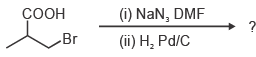Solution:
QUESTION: 6

The structure of (2S,3R)- 2- amino- 3- hydroxy butanoic acid is

Solution:
QUESTION: 7

The ene- yne that produces a chiral compound upon treatment with Lindlar’s catalyst is

Solution:
QUESTION: 8

An organic compound P (C4H8O) is positive to Bayer¡¦s test, but inert to sodium metal. On treatment with conc. HCl, P gives CH3CH2Cl and CH3CHO. The structure of P is

Solution:
QUESTION: 9

Which one of the following is an identity matrix?

Solution:
QUESTION: 10

The intermolecular van der Waals potential is inversely proportional to r6. The corresponding force is proportional to

Solution:
QUESTION: 11

The normal spinel among the following mixed metal- oxides is

Solution:
QUESTION: 12

The ground state term for a free ion with 3d7 configuration is

Solution:
QUESTION: 13

The reagent ‘oxine’ commonly used in analytical chemistry is

Solution:
QUESTION: 14

The species having trigonal pyramidal shape is

Solution:
QUESTION: 15

The correct statement about ionization potential (IP) is

Solution:
QUESTION: 16

Mass of one molecule of sulphuric acid is

Solution:
QUESTION: 17

The set of products formed in the following reaction is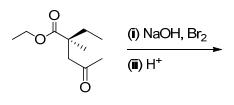Solution:
QUESTION: 18

The correct set of reagents required for the following transformation is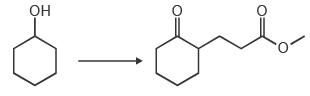Solution:
QUESTION: 19

The correct order of the pKa values for the conjugate acids of heterocyclic compounds given below is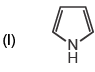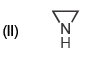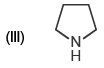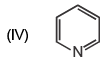Solution:
QUESTION: 20

The correct order of the 1H NMR chemical shift values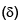for the indicated hydrogens (in bold) in the following compounds is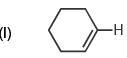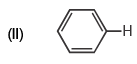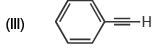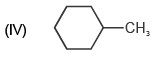Solution:
QUESTION: 21

Which of the following statements are correct for SNAr reaction?

(i) Follows second order kinetics
(ii) KH/KD > 1
(iii) Involves carbanion- type intermediate
(iv) Involves two transition states

Solution:
QUESTION: 22

According to the equipartition principle, the predicted high temperature limiting value of the molar heat capacity at constant volume for C2H2 is

Solution:
QUESTION: 23

The major product formed in the following reaction is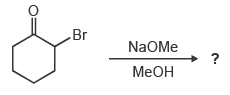Solution:
QUESTION: 24

At 25 °C, the solubility product (Ksp) of CaF2 in water is 3.2 × 10–11. The solubility (in mole per kg of water) of the salt at the same temperature (ignore ion pairing) is

Solution:
QUESTION: 25

For an isothermal free expansion of an ideal gas into vacuum, which one of the following set of values is correct?

Solution:
QUESTION: 26

The kinetics of the reaction 2N2O5 → 4NO2 + O2 in liquid bromine medium was measured independently for three different initial concentrations of N2O5: 0.11, 0.07 and 0.05 mol L–1. The half- life of the reaction was found to be 4.5 hours for all these concentrations. The order of the reaction is

Solution:
QUESTION: 27

The concentration of K+ ion inside a biological cell is 20 times higher than outside.The magnitude of potential difference between the two sides is [Given: 2.303 RT/F = 59 mV]

Solution:
QUESTION: 28

The correct order of the fundamental vibrational frequencies of the following diatomic molecules is

Solution:
QUESTION: 29

Identify the correct reagents required for the following transformation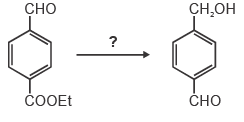Solution:
QUESTION: 30

The complex that is expected to show orbital contribution to the overall magnetic moment is

Solution:
*Multiple options can be correct
QUESTION: 31

The common feature(s) of Rb+, Kr and Br is/are that they

Solution:
*Multiple options can be correct
QUESTION: 32

The characteristics of the blue solution of sodium in liquid ammonia is/are

Solution:
*Multiple options can be correct
QUESTION: 33

Which of the following statement(s) is/are true about the transition metal- alkene complexes?

Solution:
*Multiple options can be correct
QUESTION: 34

Which of the following statement(s) is/are true about the reaction given below?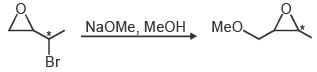Solution:
*Multiple options can be correct
QUESTION: 35

The reaction(s) which give(s) phenol is/are

Solution:
*Multiple options can be correct
QUESTION: 36

Which of the following species is/are aromatic in nature?

Solution:
*Multiple options can be correct
QUESTION: 37

Which of the following compound(s) show(s) only two signals in 1H NMR and a strong IR band at ~1690 cm–1?

Solution:
*Multiple options can be correct
QUESTION: 38

If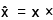and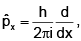then the value(s) of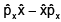is/are

Solution:
*Multiple options can be correct
QUESTION: 39

At what angle(s) of incidence, X- rays of wavelength 5.0 Å will produce diffracted beam from the (110) planes in a simple cubic lattice with a = 10 Å?

Solution:
*Multiple options can be correct
QUESTION: 40

Which of the following thermodynamic relation(s) is/are correct?

Solution:
*Answer can only contain numeric values
QUESTION: 41

Given that the crystal field stabilization energy for [Co(H2O)6]2+ is 7360 cm–1, the calculated value of Δo in kJ mol-1 is _______

Solution:
*Answer can only contain numeric values
QUESTION: 42

The amount (in grams) of potassium dichromate (MW = 294) present in 75 mL of 0.16 M aqueous solution is _______

Solution:
*Answer can only contain numeric values
QUESTION: 43

Given that the expected spin- only magnetic moment for (Et4N)2[NiCl4] is 2.83 μB, the total number of unpaired electrons in this complex is _______

Solution:
*Answer can only contain numeric values
QUESTION: 44

The pKa values of lysine are 2.18, 8.95 and 10.79. The isoelectric point of lysine is _______

Solution:
*Answer can only contain numeric values
QUESTION: 45

The amount (in grams) of NaOH (MW = 40) required for complete neutralization of one mole of the following compound is _______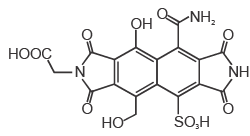Solution:
*Answer can only contain numeric values
QUESTION: 46

In the given list, the total number of compounds that form a clear homogenous solution on treatment with cold dilute H2SO4 is _______

1-propyne
cyclohexanone
cyclohexane
1-propene
propan-1-amine
propoxypropane
tetrahydropyran
ethyl butanoate
pyridine

Solution:
*Answer can only contain numeric values
QUESTION: 47

In the gas phase, the ratio of excluded volume to molecular volume for a spherical molecule is _______

Solution:
*Answer can only contain numeric values
QUESTION: 48

The pKa values of H3PO4 are 2.12, 7.21 and 12.67. The pH of a phosphate buffer containing 0.2 M NaH2PO4 and 0.1 M Na2HPO4 is _______

Solution:
*Answer can only contain numeric values
QUESTION: 49

For the reaction,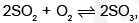the equilibrium constant KP = 5.0 at 207 °C. If the partial pressures of SO2, O2 and SO3 are 1.0 × 10–3, 0.20 and 1.0 × 10–4, respectively, then the Gibbs free energy of the reaction (ΔrG) in kJ mol-1 at 207°C is _________
[Given: R = 8.314 J K-1mol–1]

Solution:
*Answer can only contain numeric values
QUESTION: 50

Two moles of an ideal gas is expanded isothermally and reversibly from 5 to 1 bar at 298 K. The change in the entropy (in J K–1) of the system is _______

Solution:
*Answer can only contain numeric values
QUESTION: 51

The ionic radii of Cs+ and Cl- ions are 181 and 167 pm, respectively. The Born exponents for the He, Ne, Ar, Kr and Xe configurations are 5, 7, 9, 10 and 12, respectively. If the value of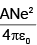is 2.45 × 10–4 J m, the lattice energy (in kJ mol–1) of CsCl according to Born- Landé equation is ________

Solution:
*Answer can only contain numeric values
QUESTION: 52

A 2.5 × 10–4 M solution of a complex exhibits an absorption maximum at 625 nm with an absorbance of 0.90 when measured in a cuvette with a path length of 1.5 cm. The absorbance of 1.5 × 10–3 M solution of the same complex recorded in a cuvette with a path length of 0.2 cm is _______

Solution:
*Answer can only contain numeric values
QUESTION: 53

A wood specimen containing 14C taken from an ancient palace showed 24 counts in 3 minutes per gram of carbon in a detector. However, a fresh wood showed 52 counts in 2 minutes per gram of carbon. Assuming no background signal in the detector and half life of 14C as 5730 years, the age (in years) of the wood specimen is _______

Solution:
*Answer can only contain numeric values
QUESTION: 54

The magnetic field (in Tesla) required for flipping a 1H nucleus in an NMR spectrometer operating at 400 MHz is _______ [Given: g = 2.67 × 108 T–1 s–1, π = 3.14]

Solution:
*Answer can only contain numeric values
QUESTION: 55

The total number of compounds (shown below) that form phenylhydrazone derivatives under acidic conditions is _______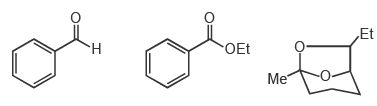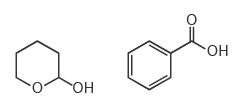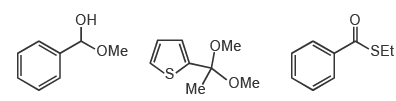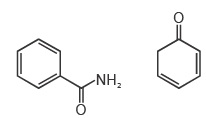Solution:
*Answer can only contain numeric values
QUESTION: 56

The number of possible monoalkylated products formed in the Friedel- Crafts reaction of anisole with 2- chloro- 3- methylbutane in the presence of anhydrous AlCl3 at 50 °C is _______

Solution:
*Answer can only contain numeric values
QUESTION: 57

For a reaction, the rate constant at 25 °C is doubled when the temperature is raised to 45 °C. The activation energy (in kJ mol–1) of the reaction is _______ [Given: ln2 = 0.693]

Solution:
*Answer can only contain numeric values
QUESTION: 58

The standard reduction potentials of the Fe3+/Fe2+ and Fe2+/Fe couples are 0.77 and –0.44 V, respectively. The standard reduction potential (in V) for the Fe3+/Fe couple is _______

Solution:
*Answer can only contain numeric values
QUESTION: 59

When a perfect monolayer of stearic acid is formed at the air- water interface, each molecule of stearic acid (MW = 284, density = 0.94 g cm–3) occupies an area of 20 Å2. The length (in Å) of the molecule is _______

Solution:
*Answer can only contain numeric values
QUESTION: 60

In an ideal monoatomic gas, the speed of sound is given by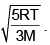If the speed of sound in argon at 25 °C is 1245 km h–1, the root mean square velocity in m s–1 is _______

Solution: